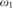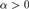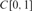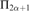Hostname: page-component-758b78586c-wkjwp Total loading time: 0 Render date: 2023-11-28T16:56:29.357Z Has data issue: false Feature Flags: { "corePageComponentGetUserInfoFromSharedSession": true, "coreDisableEcommerce": false, "useRatesEcommerce": true } hasContentIssue false

# A LIGHTFACE ANALYSIS OF THE DIFFERENTIABILITY RANK

Published online by Cambridge University Press:  17 April 2014

## Abstract

We examine the computable part of the differentiability hierarchy defined by Kechris and Woodin. In that hierarchy, the rank of a differentiable function is an ordinal less than${\omega _1}$ which measures how complex it is to verify differentiability for that function. We show that for each recursive ordinal$\alpha > 0$, the set of Turing indices of$C[0,1]$ functions that are differentiable with rank at most α is${{\rm{\Pi }}_{2\alpha + 1}}$-complete. This result is expressed in the notation of Ash and Knight.

Type
Articles
Information
The Journal of Symbolic Logic , March 2014 , pp. 240 - 265

## Access options

Get access to the full version of this content by using one of the access options below. (Log in options will check for institutional or personal access. Content may require purchase if you do not have access.)

## References

#### REFERENCES

Ash, C. J. and Knight, J., Computable structures and the hyperarithmetical hierarchy, Studies in Logic and the Foundations of Mathematics, vol. 144, North-Holland Publishing Co., Amsterdam, 2000. MR 1767842 (2001k:03090)Google Scholar
Cenzer, Douglas and Remmel, Jeffrey B., Index sets for computable differential equations. Mathematical Logic Quarterly, vol. 50 (2004), no. 4–5, pp. 329344. MR 2090381 (2005h:03083) Google Scholar
Greenberg, Noam, Montalbán, Antonio, and Slaman, Theodore A., The Slaman-Wehner theorem in higher recursion theory. Proceedings of the American Mathematical Society, vol. 139 (2011), no. 5, pp. 18651869. MR 2763773 (2012c:03119) Google Scholar
Grzegorczyk, A., On the definitions of computable real continuous functions. Fundamenta Mathematicae, vol. 44 (1957), pp. 6171. MR 0089809 (19,723c) Google Scholar
Ki, Haseo, On the Denjoy rank, the Kechris-Woodin rank and the Zalcwasser rank. Transactions of the American Mathematical Society, vol. 349 (1997), no. 7, pp. 28452870. MR 1390042 (97i:04001) Google Scholar
Kechris, Alexander S. and Hugh Woodin, W., Ranks of differentiable functions. Mathematika, vol. 33 (1986), no. 2, pp. 252278. MR 882498 (88d:03097) Google Scholar
Lacombe, Daniel, Extension de la notion de fonction récursive aux fonctions d’une ou plusieurs variables réelles. II, III. Comptes Rendus de l’Acad[start]é[end]mie des Sciences, vol. 241 (1955), pp. 1314, 151–153. MR 0072080 (17,225e) Google Scholar
Lempp, Steffen, Hyperarithmetical index sets in recursion theory. Transactions of the American Mathematical Society, vol. 303 (1987), no. 2, pp. 559583.Google Scholar
Mazurkiewicz, S., Über die Menge der differenzierbaren Funktionen.Fundamenta Mathematicae, vol. 27 (1936), pp. 244249.Google Scholar
Myhill, J., A recursive function, defined on a compact interval and having a continuous derivative that is not recursive. Michigan Mathematical Journal, vol. 18 (1971), pp. 9798. MR 0280373 (43 #6093) Google Scholar
Pour-El, Marian B. and Ian Richards, J., Computability in analysis and physics , Perspectives in Mathematical Logic, Springer-Verlag, Berlin, 1989. MR 1005942 (90k:03062) Google Scholar
Sacks, Gerald E., Higher recursion theory , Perspectives in Mathematical Logic, Springer-Verlag, Berlin, 1990. MR 1080970 (92a:03062) Google Scholar
Simpson, Stephen G., Subsystems of second order arithmetic, second ed., Perspectives in Logic, Cambridge University Press, Cambridge, 2009. MR 2517689 (2010e:03073) CrossRefGoogle Scholar
Soare, Robert I., Recursively enumerable sets and degrees , Perspectives in Mathematical Logic, Springer-Verlag, Berlin, 1987, A study of computable functions and computably generated sets. MR 882921 (88m:03003) Google Scholar
Spector, Clifford, Recursive well-orderings, this Journal, vol. 20 (1955), pp. 151–163. MR 0074347 (17,570b) Google Scholar